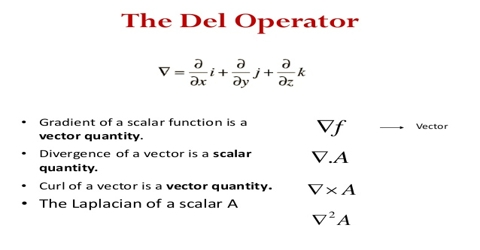# Vector Operator

Operator is an English term. It is a mathematical symbol or index. It has no value of its own. For example: square, cube, root, sine, log, etc. But when they act on or be associated with other quantities then they carry fixed values.

As for example; 32 = 9; 33 = 27, √25 = 5, sin 30° = 0.5 etc.

In other word (10 x) symbol does’ not have any value. But (10 x 5) has fixed value = 50. It means multiplication of 10 by 5. Now if (10 x) is designated by index C, then 10 x 5 = C5. So C is an operator.

Definition: The mathematical symbol or index by which one quantity can be transformed into another quantity or can explain a variable quantity, then it is called an operator.

Differentiation is an operator with respect to t. This operator is d/dt, respect to x it is d/dx, with respect to y it is d/dy etc. Integration is also an operator. Its symbol is ∫ or ∑. The vector differentiation operator is designated by symbol ∆ (del). It can be written in different components as;

∆ = î ∂/∂x + ĵ ∂/∂y + ƙ ∂/∂z

Since both scalar and vector quantities can be differentiated, so, differentiation is effective for both scalar and vector quantities.